# DEVSQ Function

Calculates the sum of the squared deviations from the sample mean

## Sum of Squared Deviations in Excel

The DEVSQ Function is categorized under Excel Statistical functions. It will calculate the sum of the squared deviations from the sample mean. The function, for example, can be used to find the variation in temperatures, blood pressure readings, etc.

As a financial analyst, DEVSQ is useful in understanding the variance in daily stock values that can signify market instability and higher risks for investors.

When we take the square root of the sum of squares, we get the standard deviation, an even more useful number. The variance and standard deviation functions deal with negative deviations by squaring deviations before they are averaged. DEVSQ calculates the sum of the squared deviations from the mean, without dividing by N or by N-1.

### Formula

=DEVSQ(number1, [number2], …)

The DEVSQ function uses the following arguments:

1. Number1 (required argument) – This is the value for which we wish to calculate the sum of squared deviations.
2. Number2 (optional argument) – A second numeric value (or array of numeric values) for which we want to calculate the sum of the squared deviation.

The arguments can be between 1 to 255. However, in Excel 2003, the DEVSQ function can only accept up to 30 number arguments. We can use a single array or a reference to an array instead of arguments, separated by commas.

The equation for the sum of squared deviations is: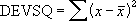### How to use the DEVSQ Function in Excel?

To understand the uses of the DEVSQ function, let’s consider an example:

#### Example

Suppose we are given the following data: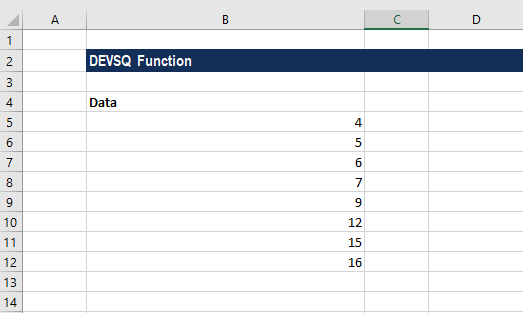The formula to use for calculating the sum of the squared deviations is: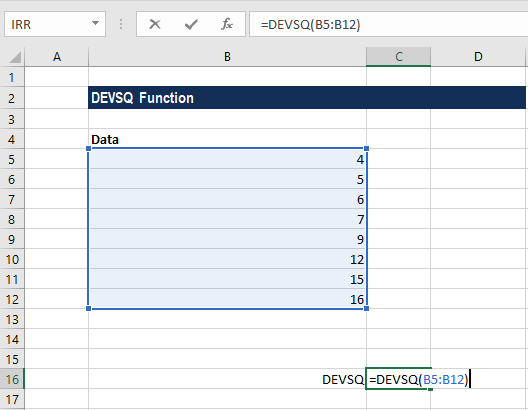We get the result below: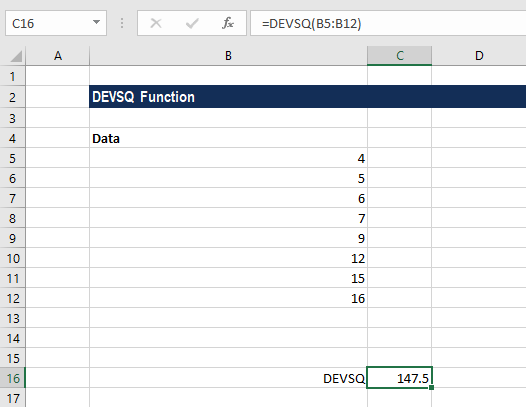### Things to remember about the DEVSQ Function

1. #VALUE! error – Occurs when any of the number arguments that are provided directly to the DEVSQ function are text strings that cannot be interpreted as numbers.
2. #NUM! error – Occurs when none of the values supplied to the DEVSQ function is numeric. Text representations of numbers that are provided as a part of an array are not interpreted as numeric values by the function.
3. For DEVSQ, arguments can be numbers, names, arrays, or references that contain numbers.
4. Empty cells and cells that contain text or logical values are ignored by the function. Hence, they will not be included in the calculation.

### Free Excel Course

To keep learning about Excel functions and developing your skills, check out our Free Excel Crash Course! Learn how to create more sophisticated financial analysis and modeling and become a successful financial analyst.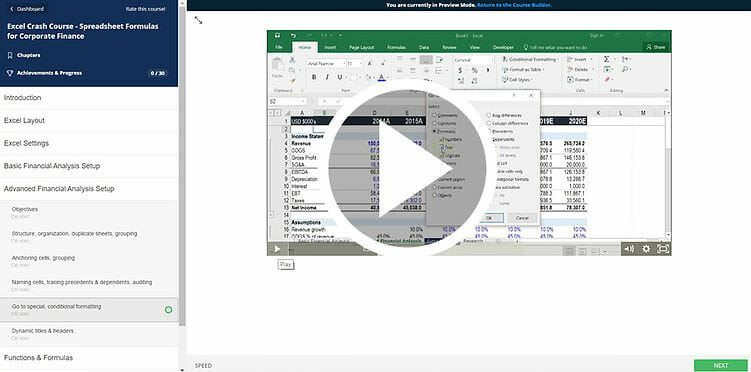Thanks for reading CFI’s guide to the Excel DEVSQ function. By taking the time to learn and master these functions, you’ll significantly speed up your financial analysis. To learn more, check out these additional CFI resources:

• Excel Functions for Finance